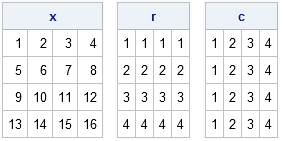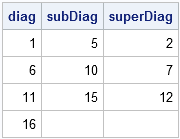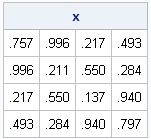Sometimes in matrix computations you need to obtain the values of certain submatrices such as the diagonal elements or the super- or subdiagonal elements. About a year ago, I showed one way to do that: convert subscripts to indices and vice-versa.

However, a tip from @RLangTip on Twitter got me thinking about a different way to solve the same problem. The tweet was the following:

A single index can be used to select from a matrix. For example, X[row(X)==col(X)] is the same as diag(X) http://t.co/EY1uMfny

I was not familiar with the row or col functions in R, but they return a matrix that is the same size as X, but with integer values that correspond to the row (column) of X. In the SAS/IML language, you can define these functions as follows:

```proc iml; /* return matrix m such that m[i,j] = j */ start col(x); return( repeat(1:ncol(x), nrow(x)) ); finish; /* return matrix m such that m[i,j] = i */ start row(x); return( repeat( T(1:nrow(x)), 1, ncol(x) )); finish;   x=shape(1:16, 4); /* define 4x4 matrix */ r = row(x); c = col(x); print x, r, c;```Update: The ROW function and COL function are distributed with SAS/IML 12.3, which was released with SAS 9.4.

When I started thinking about these matrices, I realized that they can be used to assign, extract, and copy super- and subdiagonal elements, as well as upper and lower triangular portions of a matrix. For example, the following statements extract the diagonal, the superdiagonal, and the subdiagonal elements of the matrix x:

```/* find indices of diagonal, superdiagonal, and subdiagonal */ diagIdx = loc(r=c); superIdx = loc(r=c-1); subIdx = loc(r=c+1);   /* extract elements */ diag = x[diagIdx]; superDiag = x[superIdx]; subDiag = x[subIdx]; print diag subDiag superDiag;```You can also use the diagIdx, superIdx, and subIdx vectors to assign values to the x matrix. For example,
x[diagIdx]=1:4;
assigns the values 1, 2, 3, and 4 to the diagonal elements of x.

You can also use the ROW and COL functions to extract or assign values for the upper or lower triangular portions of a matrix. For example, the following statements fill a matrix with uniform random numbers and copy values from the lower triangular portion into the upper triangular portion, which results in a random symmetric matrix:

```/* create random symmetric matrix */ call randseed(1234); x = j(4, 4); call randgen(x, "Uniform");   upperTri = loc(r <= c); /* upper tri indices in row major order */ v = vech(x); /* lower tri element in col major order */ x[uppertri] = v; /* copy elements */ print x[format=4.3];```The advantage of using this approach is its convenience. This technique makes it simple to specify elements of a banded or triangular matrix. For dealing with small matrices, I like this method and I will add it to my programming toolkit.

The disadvantage of this method is its inefficiency, especially for large matrices. The ROW and COL functions each return matrices that are the same size as x. If want to extract the diagonal of a 1000x1000 matrix, I need to create two additional matrices of the same size, plus there are further (temporary) matrices created by expressions such as r=c or r > c, which create matrices of zeros and ones. Although I like the convenience of the ROW and COL functions, for the sake of efficiency I don't plan to discard my functions that convert subscripts to indices.

ShareDistinguished Researcher in Computational Statistics

Rick Wicklin, PhD, is a distinguished researcher in computational statistics at SAS and is a principal developer of SAS/IML software. His areas of expertise include computational statistics, simulation, statistical graphics, and modern methods in statistical data analysis. Rick is author of the books Statistical Programming with SAS/IML Software and Simulating Data with SAS.

1.Hello Rick,

I want to know how can I fill a vector with the upper triangular half elements of a matrix.

So I have a matrix M and I want to write a column vector v(M) such that the element at the (i,j)th location (j>=i) in this upper triangular half is the l-th element of the vector v(M) where l=(i-1)[p-(i/2)]+j, j>=i.

Thanks.

•You can use the VECH function on the transpose:
v = vech(M`);

You could also use the ideas in this post:
idx = loc(row(M)< =col(M));
v = M[idx];

2.Osmel Brito Bigott on

Hi Rick, excelent post

Is it possible to do this without IML? maybe using arrays?

•You can use arrays and nested DO loops in the DATA step to write a data set that looks like a banded matrix.